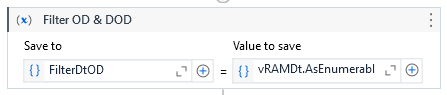# Filter Data Table from excel

Hey Im getting this error : The source contains no DataRows.
I am using this filter expression : Dt.AsEnumerable.Where(Function(row) row(vDateNow).equals(“A”) OrElse row(vDateNow).Equals(“B”)).CopyToDataTable

How to handle the error

Hi @ainaasyhda ,
you use LINQ to filter data table, can you share your sample?
regards,

Hi i using this LIINQ expressionvRAMDt.AsEnumerable.Where(Function(row) row(vDateNow).equals(“DOD”) OrElse row(vDateNow).Equals(“OD”)).CopyToDataTable

Im getting error when the filtered data is not there. how to handle this?

Hello @ainaasyhda

Assuming you have a DataTable named ‘Dt’ and a variable ‘vDateNow’ for the column name

Filter the DataTable using LINQ and store the result in a variable

Dim filteredRows = Dt.AsEnumerable().Where(Function(row) row(vDateNow).Equals(“A”) OrElse row(vDateNow).Equals(“B”))

Check if there are matching rows
If filteredRows.Any() Then
’ If there are matching rows, copy them to a new DataTable
Dim filteredDataTable = filteredRows.CopyToDataTable()
’ Now, ‘filteredDataTable’ contains the filtered rows
Else
’ Handle the case where no matching rows were found
End If

Thanks & Cheers!!!

Hi for this i need to use “IF ELSE” activity right? what condition should i write?
Sorry ! im a beginner.
Thank you for the help !!

I mean FilteredRows is a datable, how can i put the variable in IF condition?

@ainaasyhda

Use IF Activity & Didnt write any condition in ELSE…

If you are using the above expression directly to assign some rows, please use an if condition first
Check if

Dt.AsEnumerable.Where(Function(row) row(vDateNow).equals(“A”) OrElse row(vDateNow).Equals(“B”)).Count>0

in the Then sequence use an assign activity with

newDT=Dt.AsEnumerable.Where(Function(row) row(vDateNow).equals(“A”) OrElse row(vDateNow).Equals(“B”)).CopyToDataTable

in the else section you can log saying no rows are found with criteria.

The above method would work.

Thanks

Hiiii,

Its working now! thank you so muchh for your help!
i give u 5 stars !!!Cheers !!!

1 Like

Thanks a lot @ainaasyhdaThis topic was automatically closed 3 days after the last reply. New replies are no longer allowed.